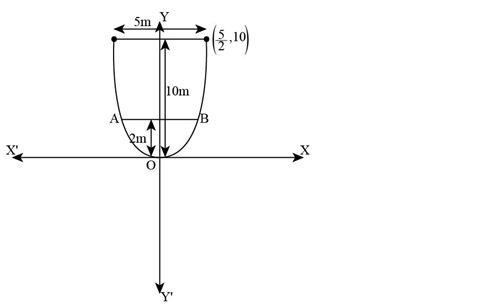An arch is in the form of a parabola with its axis vertical. The arch is 10 m high and 5 m wide at the base. How wide is it 2 m from the vertex of the parabola?

Asked by Pragya Singh | 1 year ago |  79

##### Solution :-

We know that the origin of the coordinate plane is taken at the vertex of the arch, where its vertical axis is along the positive y –axis.

Diagrammatic representation is as follows:The equation of the parabola is of the form x2 = 4ay (as it is opening upwards).

It is given that at base arch is 10m high and 5m wide.

So, y = 10 and x = $$\dfrac{5}{2}$$ from the above figure.

It is clear that the parabola passes through point ($$\dfrac{5}{2}$$, 10)

So, x2 = 4ay

$$(\dfrac{5}{2})^2$$ = 4a(10)

4a = $$\dfrac{25}{(4×10)}$$

a = $$\dfrac{5}{32}$$

we know the arch is in the form of a parabola whose equation is

$$x^2=\dfrac{5}{8}y$$

We need to find width, when height = 2m.

To find x, when y = 2.

When, y = 2,

x2 = $$\dfrac{5}{8}(2)$$

$$\dfrac{5}{4}$$

x = $$\sqrt{\dfrac{5}{4}}$$

$$\sqrt{\dfrac{5}{2}}$$

AB = 2 × $$\sqrt{\dfrac{5}{2}}$$m

$$\sqrt{5}m$$

= 2.23m (approx.)

Hence, when the arch is 2m from the vertex of the parabola, its width is approximately 2.23m.

Answered by Abhisek | 1 year ago

### Related Questions

#### An equilateral triangle is inscribed in the parabola y2 = 4ax,

An equilateral triangle is inscribed in the parabola y2 = 4ax, where one vertex is at the vertex of the parabola. Find the length of the side of the triangle.

#### A man running a racecourse notes that the sum of the distances from the two flag posts from him is always

A man running a racecourse notes that the sum of the distances from the two flag posts from him is always 10 m and the distance between the flag posts is 8 m. Find the equation of the posts traced by the man.

#### Find the area of the triangle formed by the lines joining the vertex of the parabola x2 = 12y to the ends

Find the area of the triangle formed by the lines joining the vertex of the parabola x2 = 12y to the ends of its latus rectum.